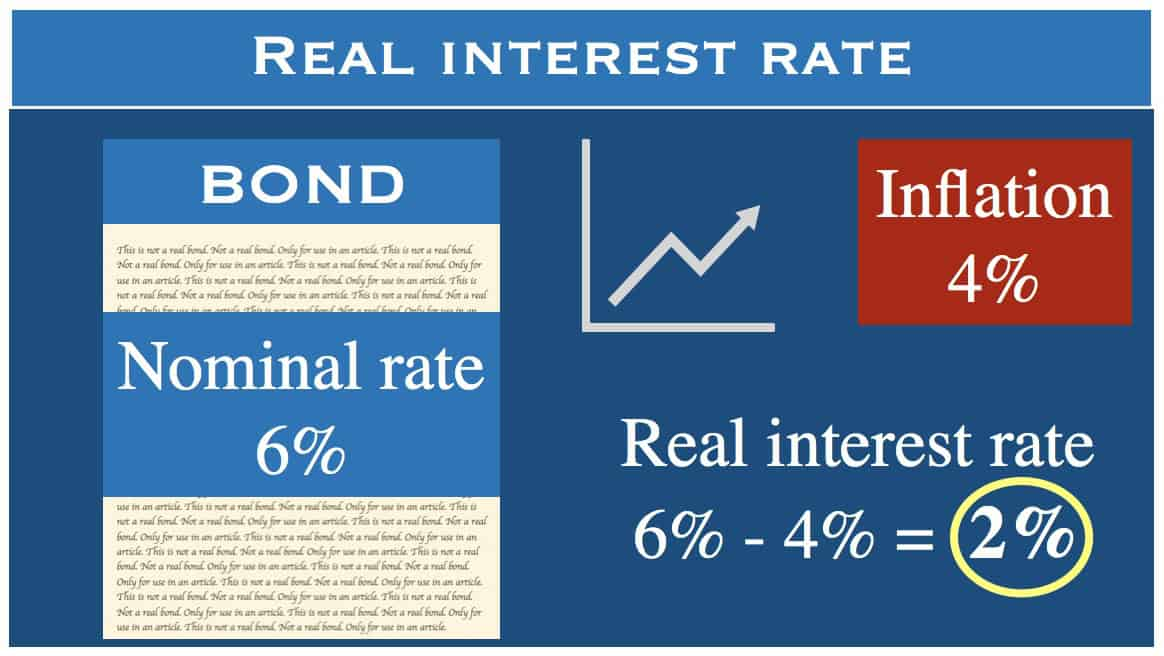# What is real interest rate? Definition and example

Real interest rate is equal to the nominal interest rate (for an investment or loan) minus the inflation rate.

It is a way of calculating interest and adjusting it to account for the economic effects of inflation. factoring in inflation allows you to keep track of the purchasing power of a specific amount of capital constant.

For example, if an investor is going to receive a 3% interest rate for their investments over the next year and there is going to be a 1% rise in prices (inflation), then the real interest rate he or she would receive would be 2%.The real interest rate is lower than the nominal interest rate, unless there is deflation.

It is important to note when using real interest rates that inflation can differ depending on the country and that it can also change significantly during a fiscal year.

Interest rates tend to be greater when the inflation rate is high, and lower when prices rise less (or not at all).

Therefore, real interest rate is a more accurate depiction of the stability of an economy compared to the nominal interest rate.

“The actual interest rate (the nominal interest rate) minus the inflation rate. Real interest rates are considered when any monetary transaction has time factored in – for example you lend money to someone for a year and ask to be repaid the principal plus interest of 5 per cent.”

## The Fisher equation

The relationship between real interest rate, nominal interest rate and the predicted inflation rate is represented by the Fisher equation:

1+i = (1+r) (1+πe)

where:

i = nominal interest rate;
r = real interest rate;
π = expected inflation rate.

### Real interest rates, savings and consumption

When real interest rates are high, money tends to move from consumption to savings. However, when real interest rates are low, demand will shift from savings to investment and consumption.